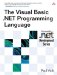# Fundamental Types

 <  Day Day Up  >

The Visual Basic .NET language predefines fundamental types that can be used to perform numeric, Boolean, and text operations (see Table 1-1). The fundamental numeric types split into three categories: integers of varying ranges ( Byte , Short , Integer , and Long ), floating-point numbers of varying ranges and precision ( Single , Double ), and a decimal number with a fixed range and precision ( Decimal ).

##### Table 1-1. Fundamental Types

Type

Description

Boolean

A Boolean (true/false) value

Byte

A 1-byte unsigned integer

Short

A 2-byte signed integer

Integer

A 4-byte signed integer

Long

An 8-byte signed integer

Decimal

A 16-byte scaled decimal number

Single

A 2-byte single precision floating-point number

Double

A 4-byte double precision floating-point number

Date

A time/date value

Char

A single Unicode character

String

A string of Unicode characters

The language defines operators that can be used to perform standard arithmetic operations on the numeric types (see Table 1-2).

The Boolean type, which represents a true/false value, can be used to express the result of conditional expressions. The logical operators are listed in Table 1-3.

The comparison operators listed in Table 1-4 can also be used to form comparison expressions.

The String and Char types represent Unicode strings and characters, respectively. The string operators are listed in Table 1-5.

String comparisons are special in that they can be evaluated in one of two ways. If the Option Compare Binary statement is specified at the beginning of a file, strings are compared using a code-point comparison. That is, two strings match if the value of each corresponding Unicode character in the two strings matches exactly. If the Option Compare Text statement is specified at the beginning of a file, strings are compared using a locale-sensitive comparison. That is, two strings match if each character is considered equivalent in the current Framework culture at runtime. In some cultures, two different Unicode values may represent the same character, so a text comparison may produce a different result than a binary comparison. If no Option Compare statement is specified at the top of a file, the default is Option Compare Binary .

##### Table 1-2. Numeric Operators

Operator

Description

+

Subtraction and negation

*

Multiplication

/

Floating-point division

\

Integer division

Mod

Integer remainder

^

Exponentiation

Not

Bitwise NOT

And

Bitwise AND

Or

Bitwise OR

Xor

Bitwise Exclusive OR

##### Table 1-3. Logical Operators

Operator

Description

Not

Logical NOT

And

Logical AND

Or

Logical OR

Xor

Logical Exclusive OR

AndAlso

Short-circuiting logical AND

OrElse

Short-circuiting logical OR

##### Table 1-4. Comparison Operators

Operator

Description

=

Equality

<>

Inequality

<

Less than

>

Greater than

<=

Less than or equal to

>=

Greater than or equal to

##### Table 1-5. String Operators

Operator

Description

&

Concatenation

Like

Pattern matching

The Date type represents date and time values. Date and time values are specified by surrounding the date or time with hash marks.

` Dim x As Date x = #8/23/1970 4:34:12 AM# x = #4/22/1972# x = #3:41:00 PM# `

The fundamental numeric types can be augmented with enumerated numeric types. Enumerated numeric types are Byte , Short , Integer , or Long types that have assigned names to specific numeric values. By default, enumerations that do not explicitly declare an underlying type are Integer . For example, the following defines an enumerated Integer type called Color that gives the name Red to the value 1 , the name Blue to the value 2 , and the name Green to the value 3 . It also defines an enumerated Byte type called SwitchValue that gives the name Off to the value and On to the value 1 .

` Enum Color   Red = 1   Blue = 2   Green = 3 End Enum Enum SwitchValue As Byte   Off   [On] End Enum `

Once an enumeration has been defined, the enumerated values can be used in place of numbers.

` Dim Background As Color Background = Color.Red If Background = Color.Green Then   Console.WriteLine("Background is green.") End If `

The conversion operators allow converting values between compatible types. Conversion operators are defined for each fundamental type (see Table 1-6), and the general conversion operator CType can be used to convert between any two types.

##### Table 1-6. Conversion Operators

Operator

Description

CBool(x)

Boolean conversion

CByte(x)

Byte conversion

CShort(x)

Short conversion

CInt(x)

Integer conversion

CLng(x)

Long conversion

CSng(x)

Single conversion

CDbl(x)

Double conversion

CDec(x)

Decimal conversion

CChar(x)

Char conversion

CStr(x)

String conversion

CDate(x)

Date conversion

CObj(x)

Object conversion

CType(x, Type)

General conversion

By default, conversions between compatible types do not require use of a conversion operator; the compiler will implicitly attempt the conversion at runtime. However, some conversions may fail at runtime ”for example, converting a Long value to Integer may fail if the Long value is out of the range of Integer . Specifying the Option Strict statement at the top of a file will require all conversions that can fail to be stated explicitly.

 <  Day Day Up  >The Visual Basic .NET Programming Language
ISBN: 0321169514
EAN: 2147483647
Year: 2004
Pages: 173
Authors: Paul Vick

Similar book on Amazon